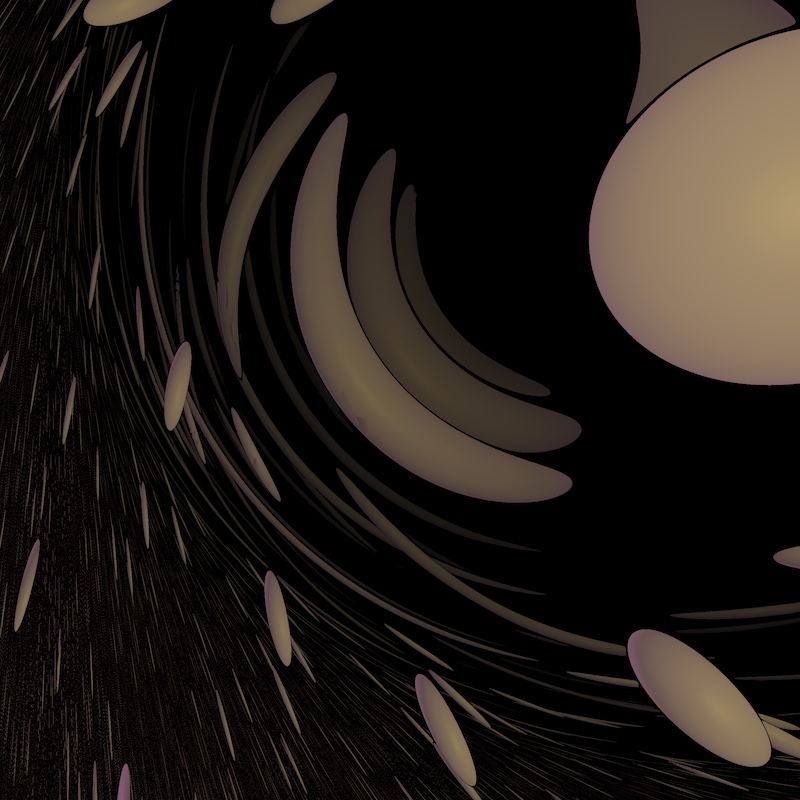# The universal cover of ${\rm SL}(2,\mathbb R)$

## What is the $\widetilde{\rm SL}(2,\mathbb R)$?

${\rm SL}(2,\mathbb R)$ is the set of all $2 \times 2$-real matrices with determinant one. The space $\widetilde{\rm SL}(2,\mathbb R)$ is its universal cover. There are several ways to think about this space. ${\rm SL}(2,\mathbb R)$ is for instance the unit tangent bundle of the hyperbolic plane $\mathbb H^2$. This point of view gives $\widetilde{\rm SL}(2,\mathbb R)$ a structure of a twisted metric line bundle over $\mathbb H^2$, which can be thought as a hyperbolic analogue of the Hopf fibration.

Click on the button below to reveal a concrete model of $\widetilde{\rm SL}(2,\mathbb R)$.

${\rm SL}(2, \mathbb R)$ acts transitively on the unit tangent bundle of $\mathbb H^2$. Hence, so does its universal cover. The orbit map provides a natural equivariant projection from $\widetilde{\rm SL}(2, \mathbb R)$ to $\mathbb H^2$ whose fibers are homeomorphic to $\mathbb R$. It turns out that, topologically, $\widetilde{\rm SL}(2, \mathbb R)$ is homeomorphic to $\mathbb H^2 \times \mathbb R$, for which a possible model is $$X = \left\{ (x,y,z,w) \in \mathbb R^4 \mid x^2 + y^2 - z^2 = -1, z> 0\right\}.$$ However, unlike in $\mathbb H^2 \times \mathbb E$ the metric is twisted. More precisely, we endow $X$ with an $\widetilde{\rm SL}(2, \mathbb R)$ invariant metric.

## Some views of $\widetilde{\rm SL}(2,\mathbb R)$

Warning: Some of the real-time simulations below requires a powerful graphic card. If your computer is not fast enough, you can reduce the size of your browser window. Click on the button below to reveal the fly commands.

The default controls to fly in the scene are the following. You can choose your keyboard in the Option controls pannel in the top right corner of the window

Command QWERTY keyboard AZERTY keyboard
Yaw left a q
Yaw right d d
Pitch up w z
Pitch down s s
Roll left q a
Roll right e e
Move forward arrow up arrow up
Move backward arrow down arrow down
Move to the left arrow left arrow left
Move the the right arrow right arrow right
Move upwards ' ù
Move downwards / =##### Balls in $\widetilde{\rm SL}(2,\mathbb R)$

A ball in the unit tangent bundle of a punctured torus

HD pictures of $\widetilde{\rm SL}(2,\mathbb R)$ can be found in the gallery